# http www comp nus edu sgcs 2100 Lecture

• Slides: 40http: //www. comp. nus. edu. sg/~cs 2100/ Lecture #17 Combinational Circuits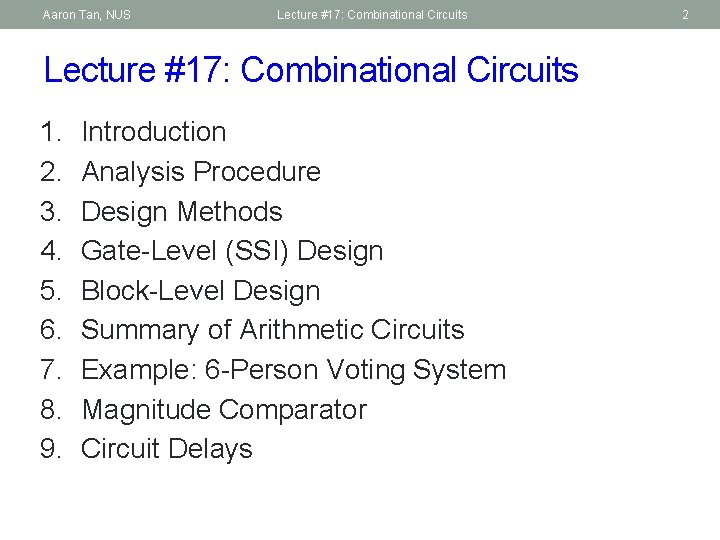Aaron Tan, NUS Lecture #17: Combinational Circuits 1. 2. 3. 4. 5. 6. 7. 8. 9. Introduction Analysis Procedure Design Methods Gate-Level (SSI) Design Block-Level Design Summary of Arithmetic Circuits Example: 6 -Person Voting System Magnitude Comparator Circuit Delays 2Aaron Tan, NUS Lecture #17: Combinational Circuits 3 1. Introduction § Two classes of logic circuits § Combinational § Sequential Circuit § Combinational Circuit § Each output depends entirely on the immediate (present) inputs : : Combinational Logic : : outputs § Each output depends on both present inputs and state. inputs : : Combinational Logic Memory : : outputsAaron Tan, NUS Lecture #17: Combinational Circuits 4 2. Analysis Procedure § Given a combinational circuit, how do you analyze its function? A B A+B What is this circuit? F 1 = (A+B). (A'+B') F 2 = (A'+B')' = A B A'+B' § Steps: 1. Label the inputs and outputs. A B F 1 F 2 0 0 0 1 1 1 1 0 0 1 2. Obtain the functions of 1 intermediate points and the outputs. 1 3. Draw the truth table. 4. Deduce the functionality of the circuit (A+B) (A'+B') Half adder.Aaron Tan, NUS Lecture #17: Combinational Circuits 3. Design Methods § Different combinational circuit design methods: § Gate-level design method (with logic gates) § Block-level design method (with functional blocks) § Design methods make use of logic gates and useful function blocks § These are available as Integrated Circuit (IC) chips. § Types of IC chips (based on packing density): SSI, MSI, LSI, VLSI, ULSI. § Main objectives of circuit design: § Reduce cost (number of gates for small circuits; number of IC packages for complex circuits) § Increase speed § Design simplicity (re-use blocks where possible) 5Aaron Tan, NUS Lecture #17: Combinational Circuits 6 4. Gate-Level (SSI) Design: Half Adder (1/2) § Design procedure: 1. State problem Example: Build a Half Adder. 2. Determine and label the inputs and outputs of circuit. Example: Two inputs and two outputs labelled, as shown below. X Y Half Adder S C (X + Y) 3. Draw the truth table. X Y C S 0 0 0 1 1 0Aaron Tan, NUS Lecture #17: Combinational Circuits 7 4. Gate-Level (SSI) Design: Half Adder (2/2) 4. Obtain simplified Boolean functions. Example: C = X Y S = X' Y + X Y' = X Y 5. Draw the logic diagram. X Y C S 0 0 0 1 1 0 Half Adder X Y Block diagram of Half Adder S X C Y Half Adder (X + Y) S CAaron Tan, NUS Lecture #17: Combinational Circuits 8 4. Gate-Level (SSI) Design: Full Adder (1/5) § Half adder adds up only two bits. § To add two binary numbers, we need to add 3 bits (including the carry). § Example: § Need Full Adder (so called as it can be made from two half adders). X Y Z Full Adder (X + Y + Z) S C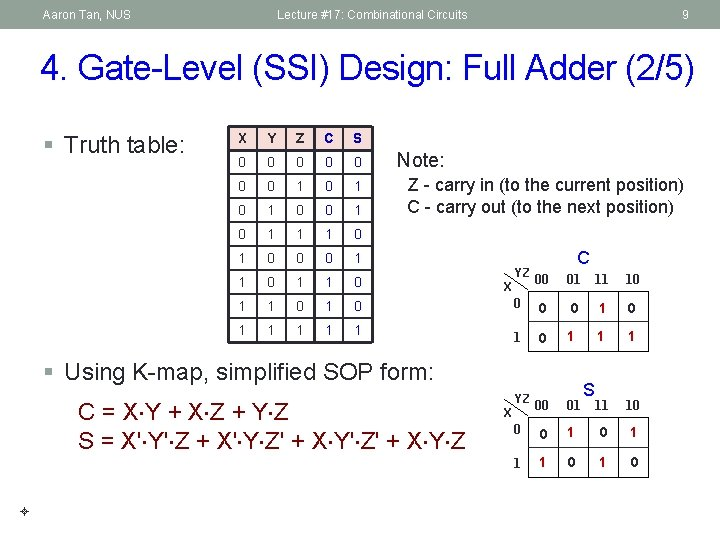Aaron Tan, NUS Lecture #17: Combinational Circuits 9 4. Gate-Level (SSI) Design: Full Adder (2/5) § Truth table: X Y Z C S 0 0 0 0 1 0 1 0 1 1 1 0 0 0 1 1 0 1 1 1 Note: Z - carry in (to the current position) C - carry out (to the next position) YZ C 00 01 11 10 0 1 0 1 1 1 00 01 11 10 0 0 1 1 1 0 X § Using K-map, simplified SOP form: C = X Y + X Z + Y Z S = X' Y' Z + X' Y Z' + X Y' Z' + X Y Z YZ X S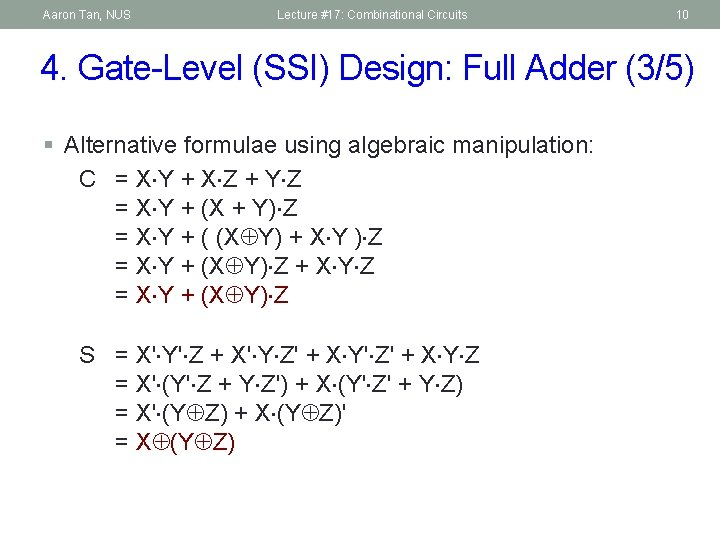Aaron Tan, NUS Lecture #17: Combinational Circuits 10 4. Gate-Level (SSI) Design: Full Adder (3/5) § Alternative formulae using algebraic manipulation: C = X Y + X Z + Y Z = X Y + (X + Y) Z = X Y + ( (X Y) + X Y ) Z = X Y + (X Y) Z + X Y Z = X Y + (X Y) Z S = X' Y' Z + X' Y Z' + X Y' Z' + X Y Z = X' (Y' Z + Y Z') + X (Y' Z' + Y Z) = X' (Y Z) + X (Y Z)' = X (Y Z)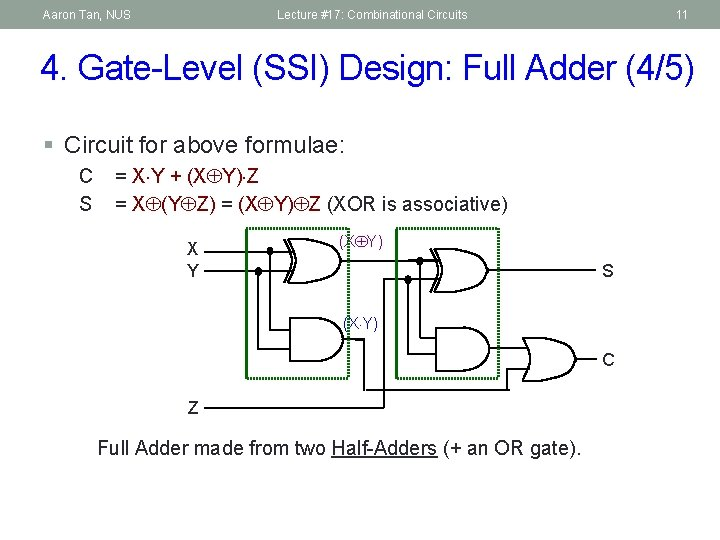Aaron Tan, NUS Lecture #17: Combinational Circuits 11 4. Gate-Level (SSI) Design: Full Adder (4/5) § Circuit for above formulae: C S = X Y + (X Y) Z = X (Y Z) = (X Y) Z (XOR is associative) X Y (X Y) S (X Y) C Z Full Adder made from two Half-Adders (+ an OR gate).Aaron Tan, NUS Lecture #17: Combinational Circuits 12 4. Gate-Level (SSI) Design: Full Adder (5/5) § Circuit for above formulae: C S Block diagrams = X Y + (X Y) Z = X (Y Z) = (X Y) Z (XOR is associative) X Y (X Y) X Y Sum Half Adder Carry (X Y) Sum S Half Adder Carry Z Full Adder made from two Half-Adders (+ an OR gate). CAaron Tan, NUS Lecture #17: Combinational Circuits 13 4. Gate-Level (SSI) Design: Code Converters § Code converter – takes an input code, translates to its equivalent output code. Input code Code converter Output code § Example: BCD to Excess-3 code converter. § Input: BCD code § Output: Excess-3 codeAaron Tan, NUS Lecture #17: Combinational Circuits 4. BCD to Excess-3 Code Converter (1/3) Digit BCD code Excess-3 code 0 0000 0011 1 0001 0100 2 0010 0101 3 0011 0110 4 0100 0111 5 0101 1000 6 0110 1001 7 0111 1010 8 1000 1011 9 1001 1100 14Aaron Tan, NUS Lecture #17: Combinational Circuits 15 4. BCD to Excess-3 Code Converter (2/3) § Truth table: § K-maps: BCD Excess-3 W A B C D W X Y Z 0 0 0 0 1 1 1 0 0 0 1 0 0 2 0 0 1 0 1 3 0 0 1 1 0 4 0 1 0 0 0 1 1 1 5 0 1 1 0 0 0 6 0 1 1 0 0 1 7 0 1 1 0 1 0 8 1 0 0 0 1 1 9 1 0 0 1 1 1 0 0 10 1 0 X X 11 1 0 1 1 X X 12 1 1 0 0 X X 13 1 1 0 1 X X 14 1 1 1 0 X X 15 1 1 X X 00 01 C 11 10 00 0 0 01 0 1 1 1 X X X 1 X X AB A CD X 11 10 1 X 00 01 C 11 10 0 1 1 1 0 0 0 11 X X 10 0 1 X X AB CD 00 B A D Y 00 01 00 1 0 01 1 0 X X 10 1 0 X X AB A D C 11 10 1 0 CD 11 D B Z AB B A CD C 11 10 00 01 00 1 01 1 0 0 1 X X 10 1 0 X X 11 D B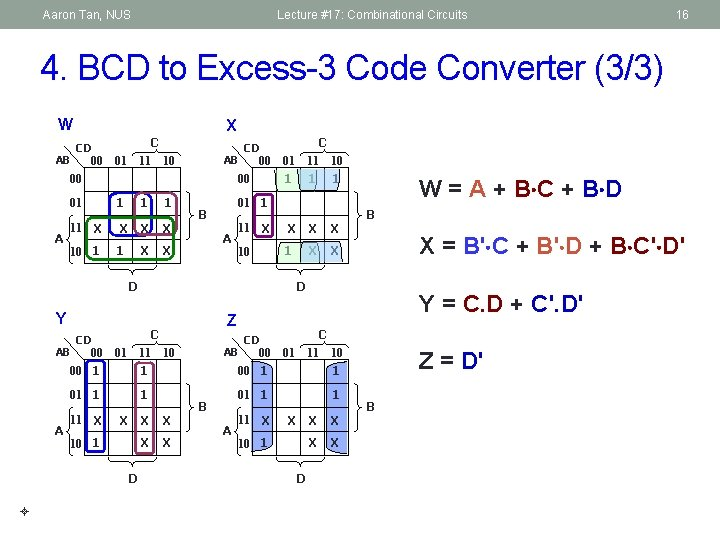Aaron Tan, NUS Lecture #17: Combinational Circuits 16 4. BCD to Excess-3 Code Converter (3/3) W AB X CD 00 C 11 10 01 AB 00 00 00 01 A CD X 11 10 1 1 X X X 1 X X 01 C 11 10 1 1 01 1 B A X 11 10 X X X 1 X X D Y AB A CD 00 00 1 1 01 1 1 11 X X 10 1 D W = A + B C + B D B X = B' C + B' D + B C' D' D Z C 11 10 01 1 X X AB B A CD 00 Y = C. D + C'. D' C 11 10 01 00 1 1 01 1 1 11 X X 10 1 D X X Z = D' B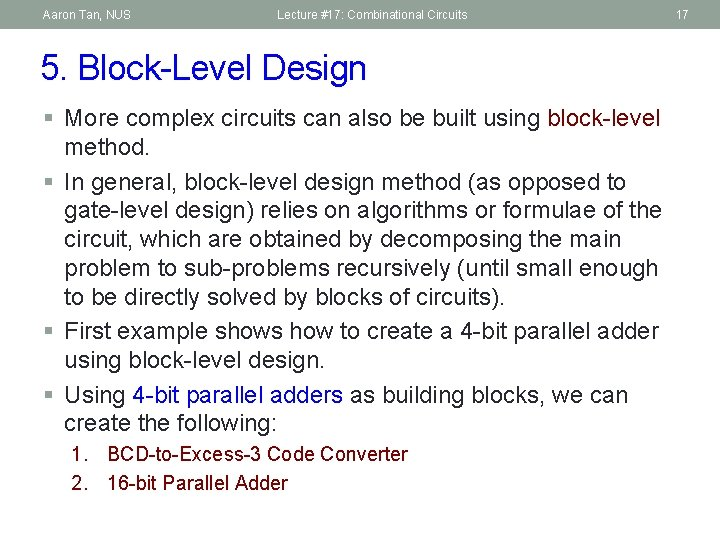Aaron Tan, NUS Lecture #17: Combinational Circuits 5. Block-Level Design § More complex circuits can also be built using block-level method. § In general, block-level design method (as opposed to gate-level design) relies on algorithms or formulae of the circuit, which are obtained by decomposing the main problem to sub-problems recursively (until small enough to be directly solved by blocks of circuits). § First example shows how to create a 4 -bit parallel adder using block-level design. § Using 4 -bit parallel adders as building blocks, we can create the following: 1. BCD-to-Excess-3 Code Converter 2. 16 -bit Parallel Adder 17Aaron Tan, NUS Lecture #17: Combinational Circuits 18 5. 4 -bit Parallel Adder (1/4) § Consider a circuit to add two 4 -bit numbers together and a carry-in, to produce a 5 -bit result. X 4 X 3 X 2 X 1 C 5 Y 4 Y 3 Y 2 Y 1 4 -bit Parallel Adder S 4 S 3 S 2 S 1 C 1 Black-box view of 4 -bit parallel adder § 5 -bit result is sufficient because the largest result is: 11112 + 12 = 111112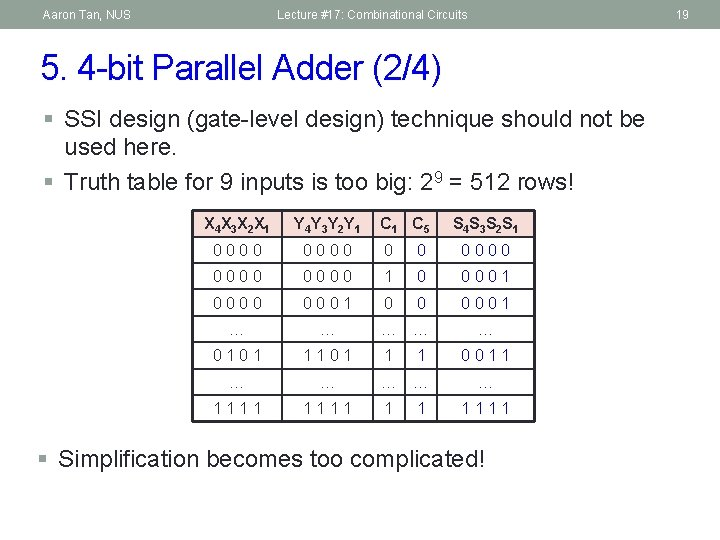Aaron Tan, NUS Lecture #17: Combinational Circuits 19 5. 4 -bit Parallel Adder (2/4) § SSI design (gate-level design) technique should not be used here. § Truth table for 9 inputs is too big: 29 = 512 rows! X 4 X 3 X 2 X 1 Y 4 Y 3 Y 2 Y 1 C 5 S 4 S 3 S 2 S 1 0000 0 0 0000 1 0 0001 0000 0001 0 0 0001 … … … 0101 1 1 0011 … … … 1111 1 1 1111 § Simplification becomes too complicated!Aaron Tan, NUS Lecture #17: Combinational Circuits 5. 4 -bit Parallel Adder (3/4) § Alternative design possible. § Addition formula for each pair of bits (with carry in), Ci+1 Si = Xi + Yi + Ci has the same function as a full adder: Ci+1 = Xi Yi + (Xi Yi) Ci Si = X i Y i Ci C= 11 0 0 X= 1010 Y= 1111 X + Y = 11 0 0 1 20Aaron Tan, NUS Lecture #17: Combinational Circuits 21 5. 4 -bit Parallel Adder (4/4) § Cascading 4 full adders via their carries, we get: Y 4 X 4 Y 3 X 3 C 4 C 5 FA S 4 Y 2 X 2 C 3 FA S 3 Y 1 X 1 C 2 FA S 1 C 1 Input Output § Note that carry is propagated by cascading the carry from one full adder to the next. § Called Parallel Adder because inputs are presented simultaneously (in parallel). Also called Ripple-Carry Adder.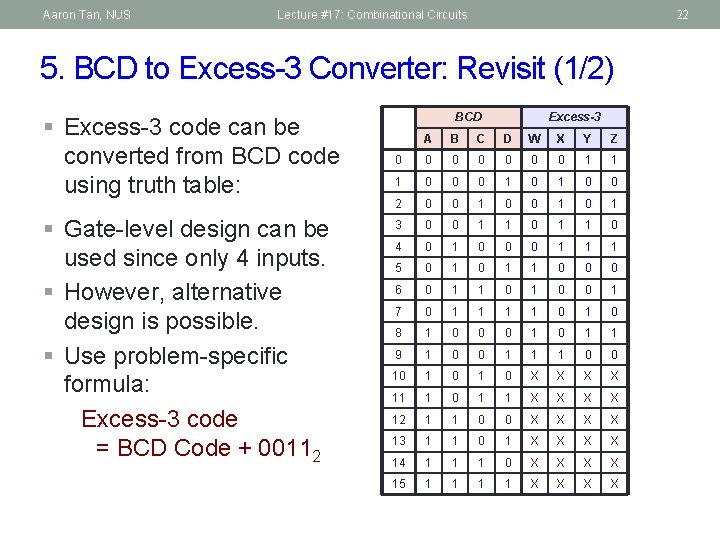Aaron Tan, NUS Lecture #17: Combinational Circuits 22 5. BCD to Excess-3 Converter: Revisit (1/2) § Excess-3 code can be converted from BCD code using truth table: § Gate-level design can be used since only 4 inputs. § However, alternative design is possible. § Use problem-specific formula: Excess-3 code = BCD Code + 00112 BCD Excess-3 A B C D W X Y Z 0 0 0 0 1 1 1 0 0 0 1 0 0 2 0 0 1 0 1 3 0 0 1 1 0 4 0 1 0 0 0 1 1 1 5 0 1 1 0 0 0 6 0 1 1 0 0 1 7 0 1 1 0 1 0 8 1 0 0 0 1 1 9 1 0 0 1 1 1 0 0 10 1 0 X X 11 1 0 1 1 X X 12 1 1 0 0 X X 13 1 1 0 1 X X 14 1 1 1 0 X X 15 1 1 X X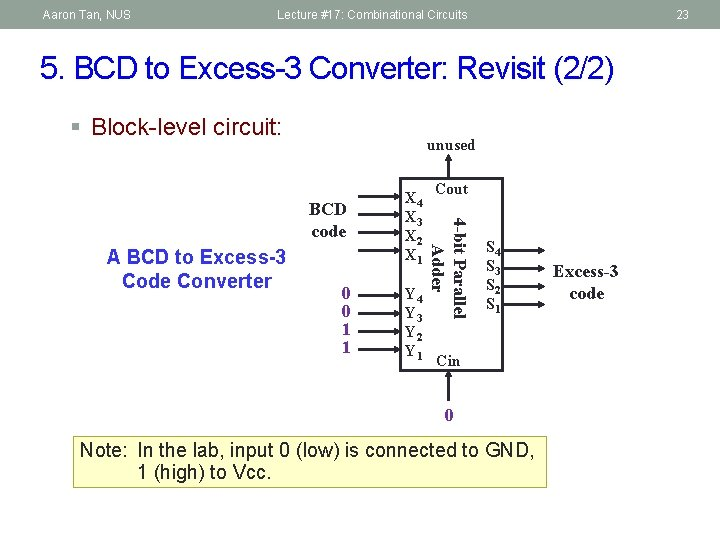Aaron Tan, NUS Lecture #17: Combinational Circuits 23 5. BCD to Excess-3 Converter: Revisit (2/2) § Block-level circuit: unused A BCD to Excess-3 Code Converter 0 0 1 1 Y 4 Y 3 Y 2 Y 1 Cout 4 -bit Parallel Adder BCD code X 4 X 3 X 2 X 1 S 4 S 3 S 2 S 1 Cin 0 Note: In the lab, input 0 (low) is connected to GND, 1 (high) to Vcc. Excess-3 codeAaron Tan, NUS Lecture #17: Combinational Circuits 24 5. 16 -bit Parallel Adder § Larger parallel adders can be built from smaller ones. § Example: A 16 -bit parallel adder can be constructed from four 4 -bit parallel adders: X 16. . X 13 Y 16. . Y 13 4 C 17 X 12. . X 9 Y 12. . Y 9 4 4 -bit // adder 4 C 13 4 S 16. . S 13 A 16 -bit parallel adder X 8. . X 5 4 4 -bit // adder 4 S 12. . S 9 Y 8. . Y 5 4 C 9 X 4. . X 1 4 4 -bit // adder Y 4. . Y 1 4 C 5 4 4 -bit // adder 4 C 1 4 S 8. . S 5 S 4. . S 1 4 S 4. . S 1 = S 4 S 3 S 2 S 1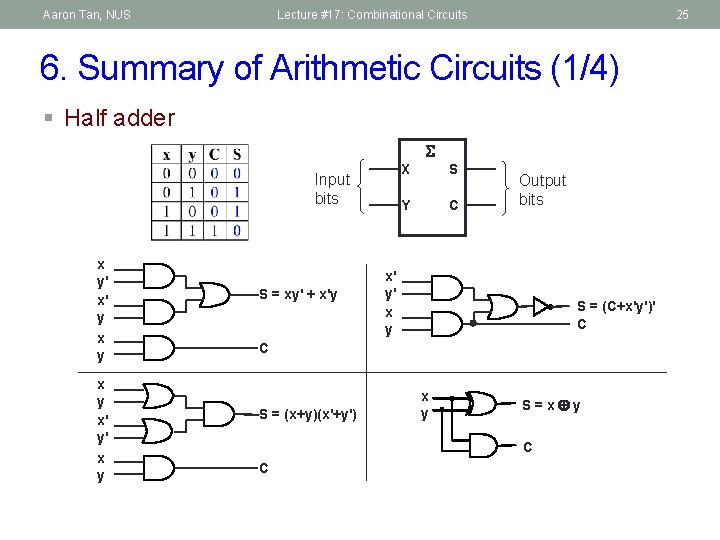Aaron Tan, NUS Lecture #17: Combinational Circuits 25 6. Summary of Arithmetic Circuits (1/4) § Half adder Input bits x y' x' y x y x' y' x y S = xy' + x'y X S Y C Output bits x' y' x y S = (C+x'y')' C C S = (x+y)(x'+y') x y S=x y C CAaron Tan, NUS Lecture #17: Combinational Circuits 26 6. Summary of Arithmetic Circuits (2/4) § Full adder A Input bits X' y' z x' y z' x y' z' x y z yz x 1 x y x z y z B Cin 1 1 1 Output bits Cout 00 01 11 10 0 S S yz x 00 01 11 10 1 C = xy + xz + yz 1 1 S = x'y'z + x'yz' + xy'z' + xyz x y S = (x y) z x y C C = x y + (x y) z z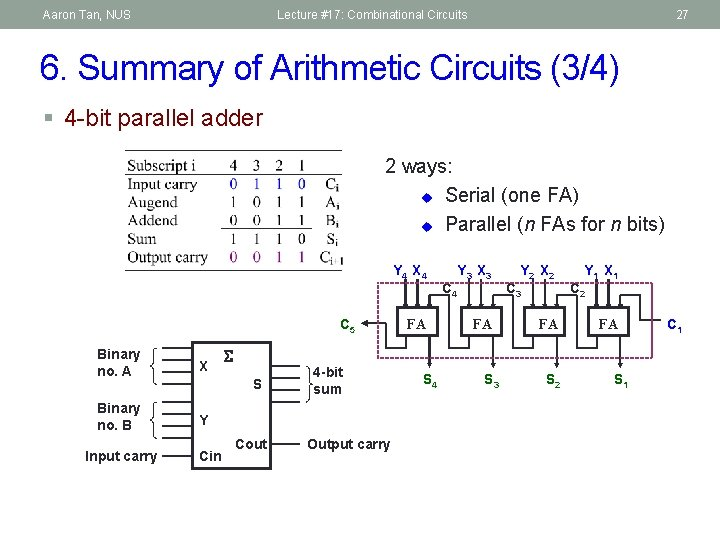Aaron Tan, NUS Lecture #17: Combinational Circuits 27 6. Summary of Arithmetic Circuits (3/4) § 4 -bit parallel adder 2 ways: u Serial (one FA) u Parallel (n FAs for n bits) Y 4 X 4 C 5 Binary no. A X Binary no. B Y Input carry S Cin Cout 4 -bit sum Output carry FA S 4 C 4 Y 3 X 3 FA S 3 Y 2 X 2 C 3 FA S 2 Y 1 X 1 C 2 FA S 1 C 1Aaron Tan, NUS Lecture #17: Combinational Circuits 28 6. Summary of Arithmetic Circuits (4/4) § Cascading 4 full adders (FAs) gives a 4 -bit parallel adder. § Classical method: 9 input variables 29 = 512 rows in truth table! § Cascading method can be extended to larger adders. § Example: 16 -bit parallel adder. X 16. . X 13 Y 16. . Y 13 4 C 17 X 12. . X 9 Y 12. . Y 9 4 4 -bit // adder 4 S 16. . S 13 4 C 13 X 8. . X 5 4 4 -bit // adder 4 S 12. . S 9 Y 8. . Y 5 4 C 9 X 4. . X 1 4 4 -bit // adder 4 S 8. . S 5 Y 4. . Y 1 4 C 5 4 4 -bit // adder 4 S 4. . S 1 C 1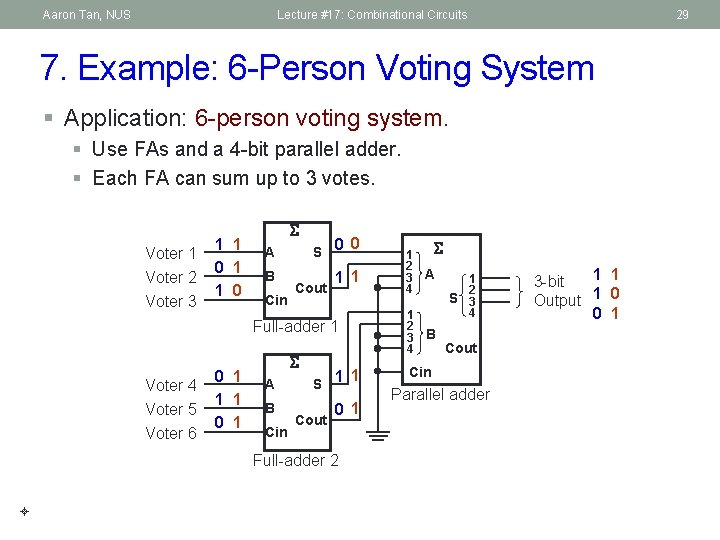Aaron Tan, NUS Lecture #17: Combinational Circuits 29 7. Example: 6 -Person Voting System § Application: 6 -person voting system. § Use FAs and a 4 -bit parallel adder. § Each FA can sum up to 3 votes. Voter 1 Voter 2 Voter 3 1 1 0 A 0 S 0 B 1 1 Cin Cout Full-adder 1 Voter 4 Voter 5 Voter 6 0 1 1 1 0 1 A B Cin 1 S 1 Cout 0 1 Full-adder 2 1 2 3 4 A 1 2 3 4 B 1 2 S 3 4 Cout Cin Parallel adder 1 1 3 -bit Output 1 0 0 1Aaron Tan, NUS Lecture #17: Combinational Circuits 8. Magnitude Comparator (1/4) § Magnitude comparator: compares 2 unsigned values A and B, to check if A>B, A=B, or A<B. § To design an n-bit magnitude comparator using classical method, it would require 22 n rows in truth table! § We shall exploit regularity in our design. § Question: How do we compare two 4 -bit unsigned values A (a 3 a 2 a 1 a 0) and B (b 3 b 2 b 1 b 0)? If (a 3 > b 3) then A > B If (a 3 < b 3) then A < B If (a 3 = b 3) then if (a 2 > b 2) … 30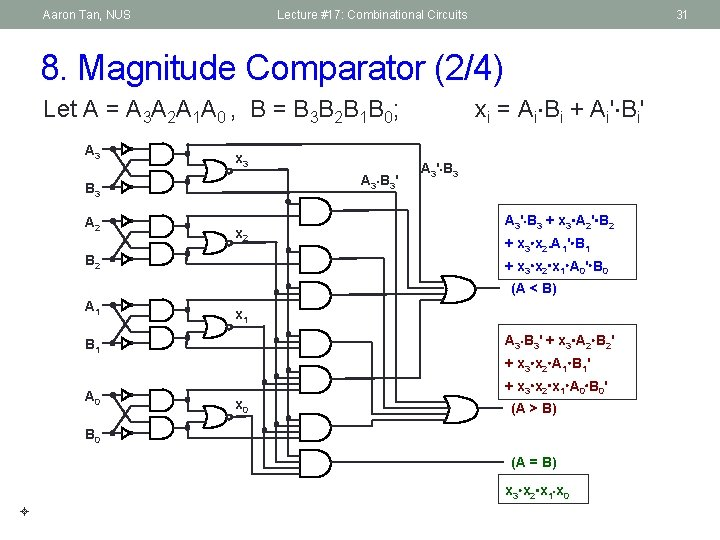Aaron Tan, NUS Lecture #17: Combinational Circuits 31 8. Magnitude Comparator (2/4) xi = Ai Bi + Ai' Bi' Let A = A 3 A 2 A 1 A 0 , B = B 3 B 2 B 1 B 0; A 3 x 3 A 3 B 3' B 3 A 2 x 2 A 3' B 3 + x 3 A 2' B 2 + x 3 x 2. A 1' B 1 + x 3 x 2 x 1 A 0' B 0 B 2 (A < B) A 1 x 1 A 3 B 3' + x 3 A 2 B 2' B 1 A 0 + x 3 x 2 A 1 B 1' + x 3 x 2 x 1 A 0 B 0' x 0 (A > B) B 0 (A = B) x 3 x 2 x 1 x 0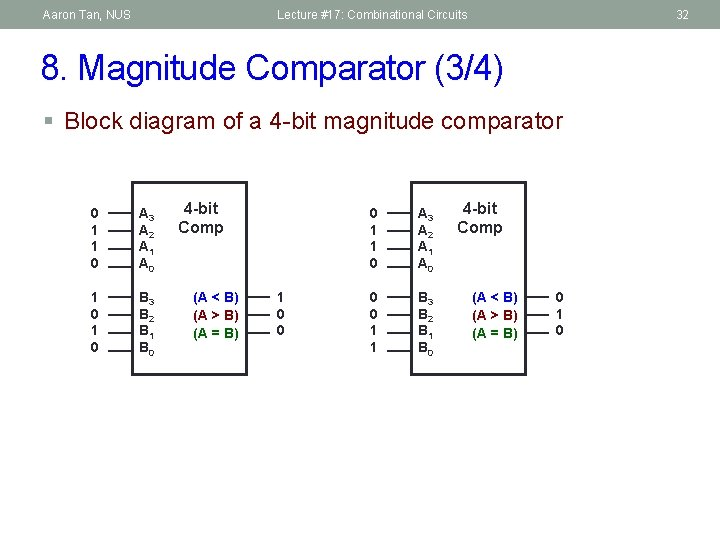Aaron Tan, NUS Lecture #17: Combinational Circuits 32 8. Magnitude Comparator (3/4) § Block diagram of a 4 -bit magnitude comparator 0 1 1 0 A 3 A 2 A 1 A 0 1 0 B 3 B 2 B 1 B 0 4 -bit Comp (A < B) (A > B) (A = B) 1 0 0 0 1 1 0 A 3 A 2 A 1 A 0 0 0 1 1 B 3 B 2 B 1 B 0 4 -bit Comp (A < B) (A > B) (A = B) 0 1 0Aaron Tan, NUS Lecture #17: Combinational Circuits 33 8. Magnitude Comparator (4/4) § A function F accepts a 4 -bit binary value ABCD, and returns 1 if 3 ABCD 12, or 0 otherwise. How would you implement F using magnitude comparators and a suitable logic gate? A B C D A 3 A 2 A 1 A 0 0 0 1 0 B 3 B 2 B 1 B 0 A 3 A 2 A 1 A 0 1 1 0 1 B 3 B 2 B 1 B 0 4 -bit Comp (A < B) (A > B) (A = B) FAaron Tan, NUS Lecture #17: Combinational Circuits 9. Circuit Delays (1/5) § Given a logic gate with delay t. If inputs are stable at times t 1, t 2, …, tn, then the earliest time in which the output will be stable is: max( t 1, t 2, …, tn ) + t t 1 t 2 : tn : Logic Gate max (t 1, t 2, . . . , tn ) + t § To calculate the delays of all outputs of a combinational circuit, repeat above rule for all gates. 34Aaron Tan, NUS Lecture #17: Combinational Circuits 35 9. Circuit Delays (2/5) § As a simple example, consider the full adder circuit where all inputs are available at time 0. Assume each gate has delay t. X Y 0 0 max(0, 0)+t = t max(t, 0)+t = 2 t S t 2 t max(t, 2 t)+t = 3 t C Z 0 § Outputs S and C experience delays of 2 t and 3 t respectively.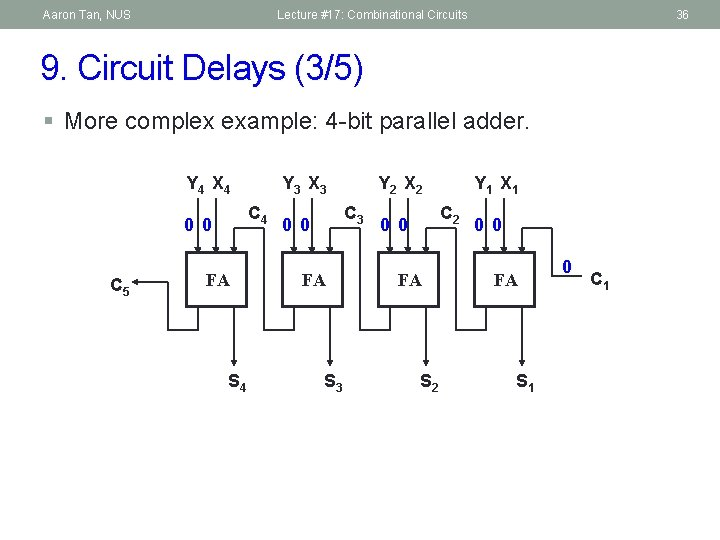Aaron Tan, NUS Lecture #17: Combinational Circuits 36 9. Circuit Delays (3/5) § More complex example: 4 -bit parallel adder. Y 4 X 4 C 4 0 0 C 5 Y 3 X 3 FA S 4 Y 2 X 2 C 3 0 0 FA S 3 Y 1 X 1 C 2 0 0 FA S 1 0 C 1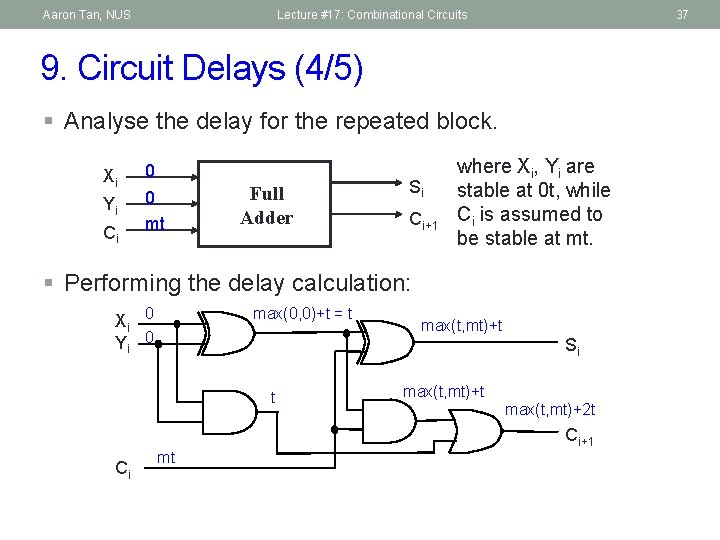Aaron Tan, NUS Lecture #17: Combinational Circuits 37 9. Circuit Delays (4/5) § Analyse the delay for the repeated block. Xi Yi Ci 0 0 mt Full Adder Si Ci+1 where Xi, Yi are stable at 0 t, while Ci is assumed to be stable at mt. § Performing the delay calculation: Xi 0 Yi 0 max(0, 0)+t = t max(t, mt)+t Si t max(t, mt)+2 t Ci+1 Ci mt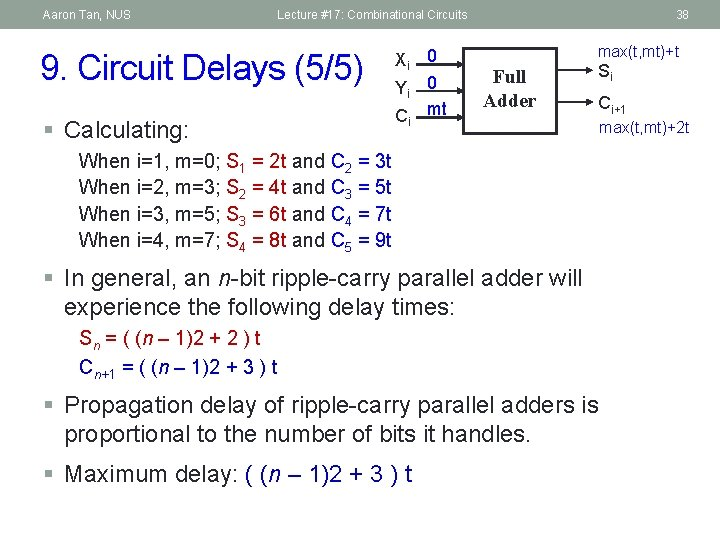Aaron Tan, NUS Lecture #17: Combinational Circuits 9. Circuit Delays (5/5) § Calculating: Xi 0 Yi 0 C mt 38 max(t, mt)+t Full Adder i Si Ci+1 When i=1, m=0; S 1 = 2 t and C 2 = 3 t When i=2, m=3; S 2 = 4 t and C 3 = 5 t When i=3, m=5; S 3 = 6 t and C 4 = 7 t When i=4, m=7; S 4 = 8 t and C 5 = 9 t § In general, an n-bit ripple-carry parallel adder will experience the following delay times: Sn = ( (n – 1)2 + 2 ) t Cn+1 = ( (n – 1)2 + 3 ) t § Propagation delay of ripple-carry parallel adders is proportional to the number of bits it handles. § Maximum delay: ( (n – 1)2 + 3 ) t max(t, mt)+2 t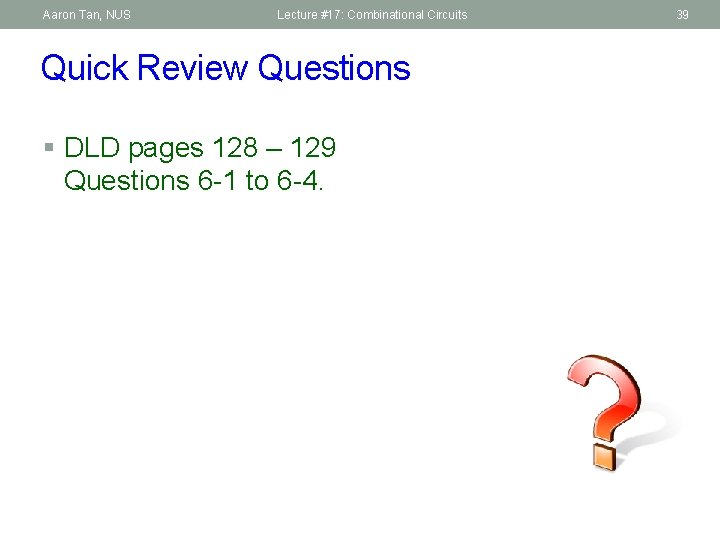Aaron Tan, NUS Lecture #17: Combinational Circuits Quick Review Questions § DLD pages 128 – 129 Questions 6 -1 to 6 -4. 39Aaron Tan, NUS Lecture #17: Combinational Circuits End of File 40